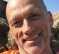Started by 2 years ago4 replieslatest reply 2 years ago189 views

In MATLAB,  there is a d2d function which resample the biquad filter directly.

Does anyone know how the d2d function works?

or the method to resample directly biquad filters?

Thank you for reading my question.

[ - ]Is it that you have some coefficients for lets say 44.1kHz filter but you don't know how they are calculated? If situation is this and those coefficients are calculated using standard methods then, this should work (Octave/Matlab code):

function [b, a] = BiquadCoefficientConverter(b, a, inFS, outFS)% inFS = original sample rate
% outFS = target sample rate
d(1)=b(1)+b(2)+b(3);d(2)=2.0*(b(1)-b(3));d(3)=b(1)-b(2)+b(3);c(1)=1+a(2)+a(3);c(2)=2.0*(1.0-a(3));c(3)=1.0-a(2)+a(3);r=outFS/inFS;invc=1/(c(1)+r*(c(2)+r*c(3)));b(1)=(d(1)+r*(d(2)+r*d(3)))*invc;b(2)=2.0*(d(1)-r^2*d(3))*invc;b(3)=(d(1)-r*(d(2)-r*d(3)))*invc;a(1)=1;a(2)=2.0*(c(1)-r^2*c(3))*invc;a(3)=(c(1)-r*(c(2)-r*c(3)))*invc;
[ - ]Thank you. I will try this.

[ - ]If you want to know/see/understand the algorithms (there are two options, ZOH and Tustin) involved in detail, you can always step thru the source code. I'm assuming of course that since you referenced the d2d function, you have access to the code. The core of the conversation algorithm is here:

~\toolbox\shared\controllib\engine\+ltipack\@zpkdata\d2d.m

But, you can always just set a breakpoint at the call site of d2d and continue to "step-in" until you hit the crux of the algorithm. It's about 15 lines and easy to read, written back in the mid 80's.

[ - ]